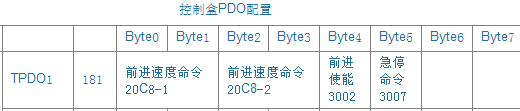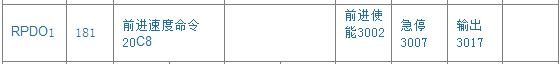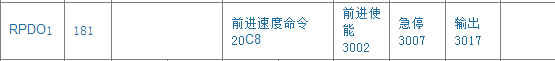# canopen PDO传输疑问1A00 = 0x20c80110
1A00 = 0x20c80210
1A00 = 0x30020008
1A00 = 0x300700081600 = 0x20c80010
1600 = 0x0
1600 = 0x30020008
1600 = 0x300700081600 = 0x0
1600 = 0x20c80010
1600 = 0x30020008
1600 = 0x30070008

20c8=0xABCD
3002=0x34
3007=0x12

20c8=0xABCD
3002=0x34
3007=0x12